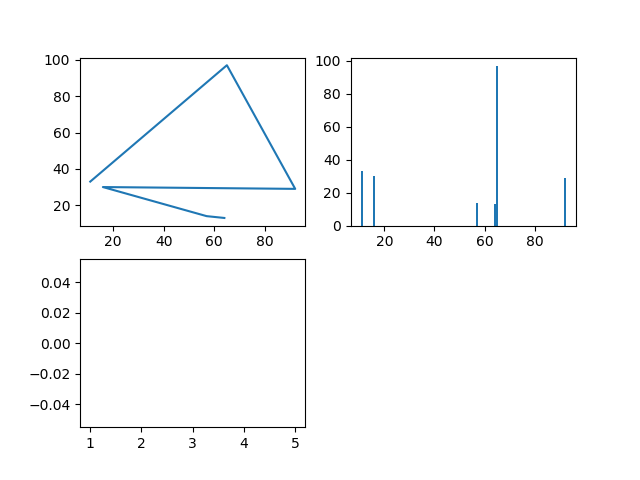•I recently just learned how to do this without using loops: [sourcecode lang=”python”] import random x = [0 for _ in range(10)] #underscore used when you do not need the value from the list. So here 0 replaces the value from range(10) #expected out put [0, 0, 0, 0, 0, 0, 0, 0, 0, 0] y = [random.random() for _ in range(20)] #similar logic here, but instead of a known value like 0, the method random generates a random value z = [random.randrange(10) for _ in range(10)] #if you want the values to be within a specific range 0 – 10 a = [random.randrange(12, 20) for _ in range(10)]#if you…

•So how do I use subplots? 08/26/2018 The code below is custom to my csv file (see below), so make sure you either use the same format or change the "get_file" function to the properly process your file. MOCK_DATA_2 The file above looks like this: x,y 64,13 57,14 16,30 92,29 65,97 11,33 [sourcecode lang=”python”] from matplotlib.pyplot import * import numpy as np, os from typing import List #get file def get_file(filename: str): #determines validity of path #returns file content filepath = os.path.abspath(filename) if os.path.exists(filepath): fh = open(filepath) header = fh.readline() #excludes first row of the csv file e.g. x,y data = {"x":[],"y":[]} for row in fh.readlines(): if len(row) &lt;= 0:…## Enjoy this blog? Please spread the word :)

error: Content is protected !!